Ex 14.3

Chapter 14 Class 10 Statistics (Term 2)
Serial order wise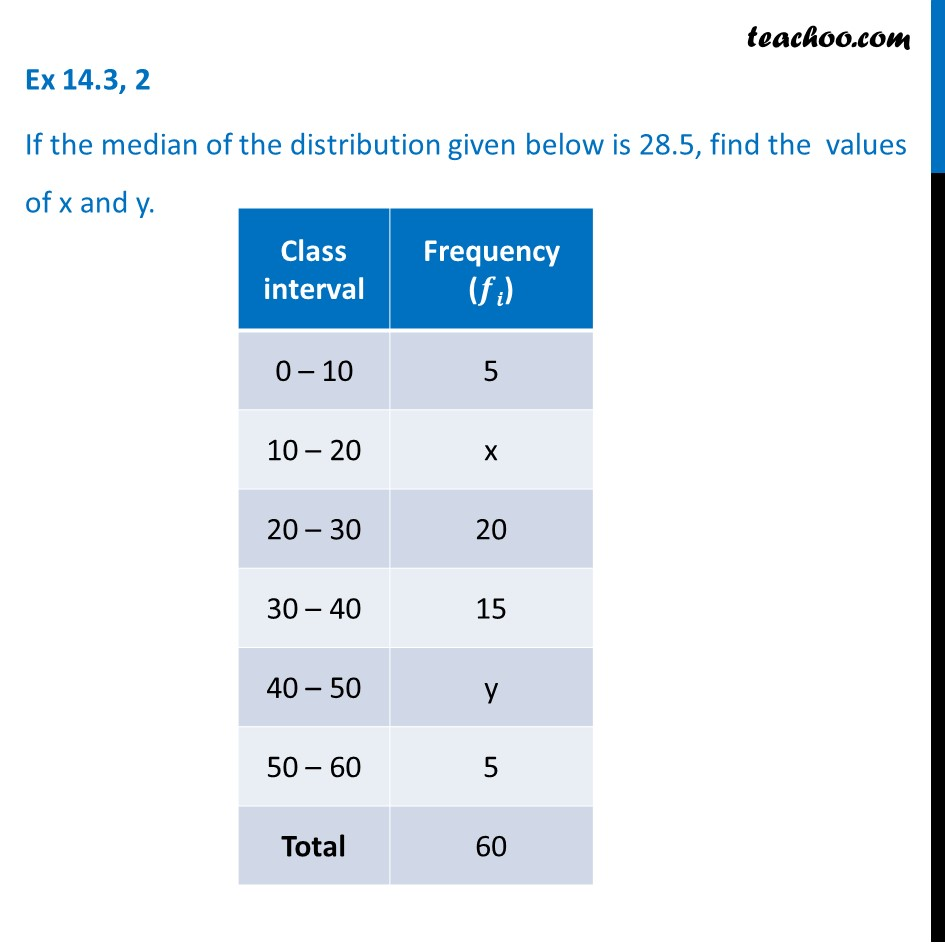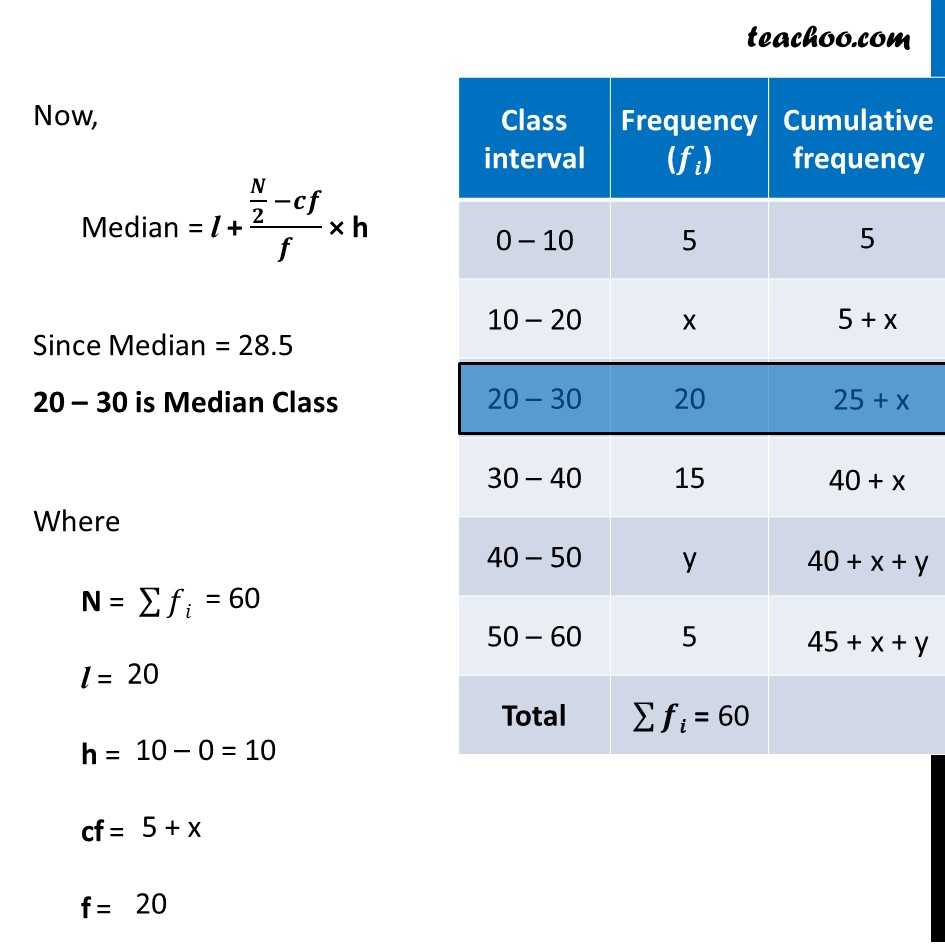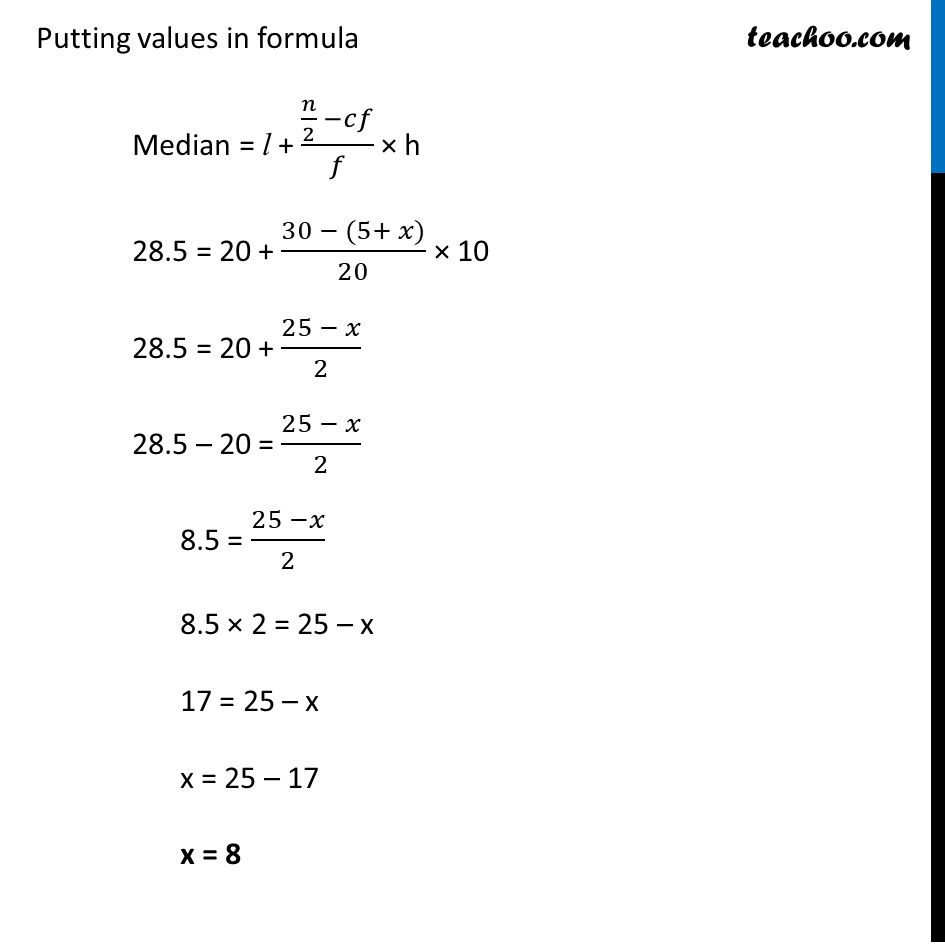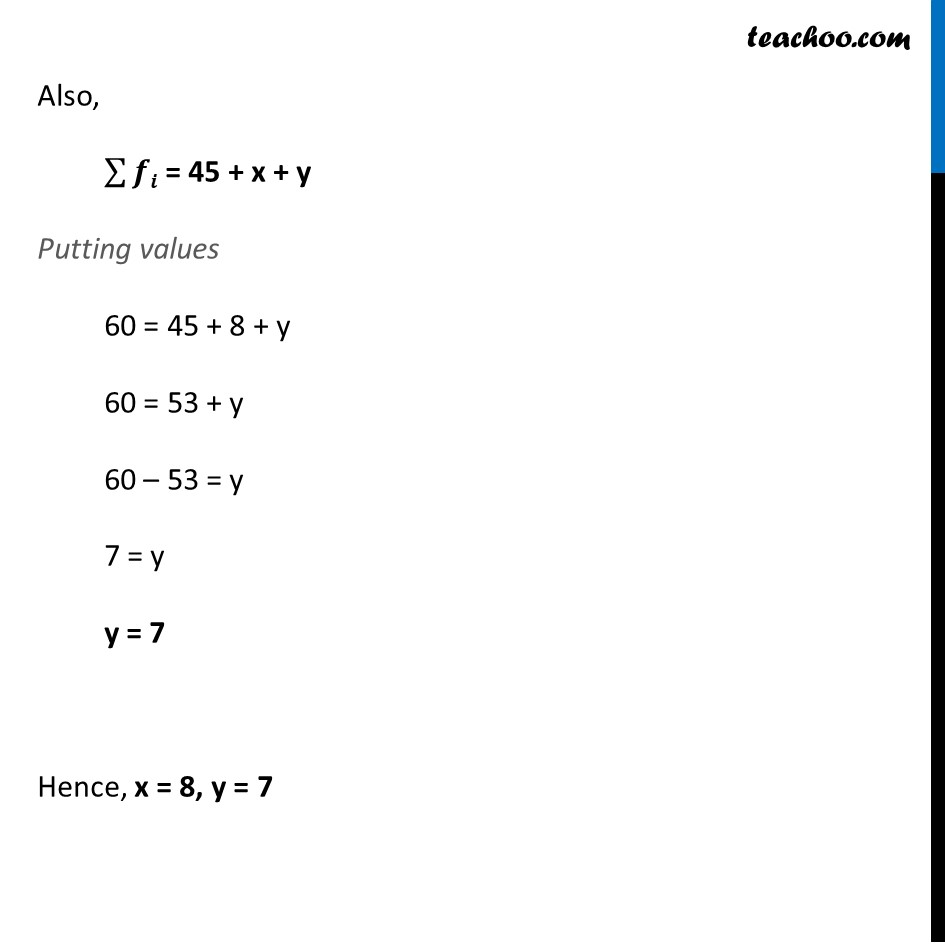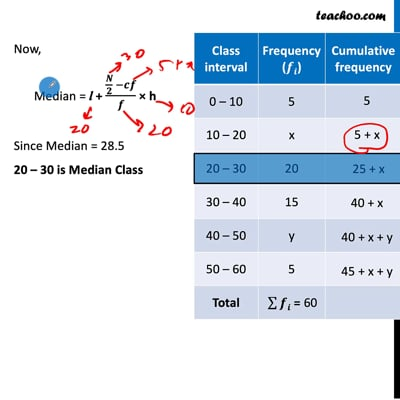This video is only available for Teachoo black users

### Transcript

Ex 14.3, 2 If the median of the distribution given below is 28.5, find the values of x and y. Now, Median = l + (𝑵/𝟐 −𝒄𝒇)/𝒇 × h Since Median = 28.5 20 – 30 is Median Class Where N = ∑▒𝑓𝑖 l = h = cf = f = Putting values in formula Median = l + (𝑛/2 −𝑐𝑓)/𝑓 × h 28.5 = 20 + (30 − (5+ 𝑥))/20 × 10 28.5 = 20 + (25 − 𝑥)/2 28.5 – 20 = (25 − 𝑥)/2 8.5 = (25 −𝑥)/2 8.5 × 2 = 25 – x 17 = 25 – x x = 25 – 17 x = 8 Also, ∑▒𝒇𝒊 = 45 + x + y Putting values 60 = 45 + 8 + y 60 = 53 + y 60 – 53 = y 7 = y y = 7 Hence, x = 8, y = 7